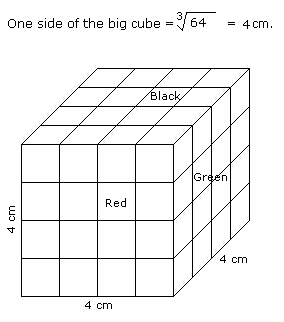# Verbal Reasoning - Cube and Cuboid

Exercise : Cube and Cuboid - Cube and Cuboid 5
Directions to Solve

The following questions are based on the information given below:

All the opposite faces of a big cube are coloured with red, black and green colours. After that is cut into 64 small equal cubes.

1.
How many small cubes are there where one face is green and other one is either black or red ?
28
8
16
24
Explanation:Number of small cubes having one face green and the other one is either red or black = 8 x 2 = 16

2.
How many small cubes are there whose no faces are coloured ?
0
4
8
16
Explanation:Number of small cubes having no face coloured = (x - 2)3

= (4 - 2)3

= 8

3.
How many small cubes are there whose 3 faces are coloured ?
4
8
16
24
Explanation:Number of small cubes having three faces coloured = 1 at each corner

= 1 x 8

= 8

>

4.
How many small cubes are there whose only one face is coloured ?
32
8
16
24
Explanation:Number of small cubes having only one face coloured = 4 from each face

= 4 x 6

= 24

5.
How many small cubes are there whose at the most two faces are coloured ?
48
56
28
24